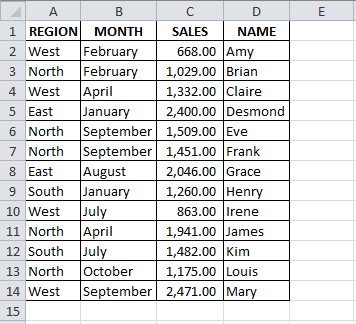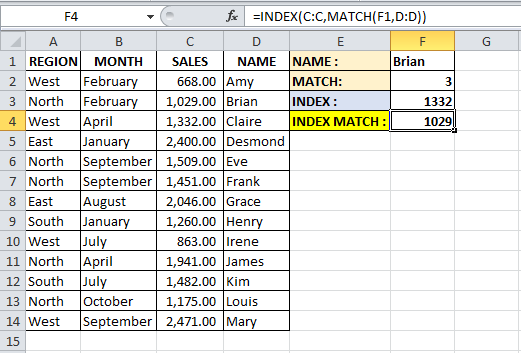# INDEX MATCH An Alternative To VLookUpHaving worked with and trained a range of people from different departments and businesses, I have seen that the VLookUp is the function that I am most asked for help with or training on.  Here I would like to show you an alternative to the VLookUp which is INDEX MATCH.

INDEX MATCH solves some of the downsides of VLookUps.

If you’re not sure what the VLookUp is like, you can read my post on VLookUp by clicking here.

You’ve probably experienced two of the downfalls of Excel that I frequently come across:

1. VLookUp works from left to right.  The first column of your table array must contain the lookup value, and the data that you want to retrieve must be to the right of this.
2. Working with smaller files, speed may not be an issue, but as the number of VLookUps increases the length of time to update the formula may increase.

The INDEX MATCH can overcome both these problems.

The data that you want to retrieve does not have to be the right of your lookup value, it can be to the left either and recently I timed the INDEX MATCH against VLookUps in the same file and the INDEX MATCH was faster by about 11% (there were over 800,000 rows of data with at least one VLookUp/INDEX MATCH in each row.

I have read that INDEX MATCH can be up to 13% faster in larger files than VLookUps.

Let’s break down the formula into it’s two formulas.  We will base our example on the data below.## MATCH

MATCH “returns the relative position of an item in an array that matches a specified value in a specified order”.

It has 3 arguments:

• Lookup_value – this is the value you use to find the value you want in the array
• Lookup_array – this is a range of cells that possibly contains your lookup value
• Match_type – this is the type of match you want.  0 indicates an exact match, 1 for the closes match less than or equal to the lookup value and -1 for the closes match that is greater than or equal to the lookup value.  If omitted, Excel uses 1 as the default.I have made a small change to our example.

In cell E1, I have entered “NAME :” and in F1 I have entered “Brian”.  We will use this cell as our lookup value later.

In E2, I have entered “MATCH :” and in F2 I have entered our Match formula as follows:

• =MATCH(
• F1, – our lookup_value will be the name in this cell
• D:D, – our lookup_array where we will look for our lookup_value
• 0) – we want to find an exact match for our looku_value

The result of the formula is 3.

The name Brian can be found in the 3rd row of our array, which was column D.

Note that if I set my array as cells D2 down to D14, the result of this formula would be 2 – that is because Brian is now in the second row of our array (D2 is first row, D3 is second row and so on).

If we change the name, the result of our formula will be the row number that the name can be found in our lookup_array.

We are going to use the MATCH formula in our final formula as a way to return the row number our lookup_value can be found in.

## INDEX

INDEX “returns a value or reference of the cell at the intersection of a particular row and column, in a given range).

There are 2 versions of the INDEX formula.  We are going to use “array, row_num, column_num” and we will use two arguments:

• Array – this is a range of cells
• Row_num – selects the row in our Array from which to return a value.

There is a 3rd argumet, Column_num which we won’t be using in this case.  But if we did not use Row_num, we would have to use Column_num.

I have made some changes to our existing file again.  In E3, I have entered “INDEX : ” and in F3, I have entered an INDEX formula.The formula can be broken down as:

• =INDEX(
• C:C, – Our array will be column C, the Sales values column.
• 3) – Our Row_num will be 3, we want to retrieve the value in the 3rd row of our array.

The result is “1029” which comes from cell C3, the 3rd row in our array.

As with the MATCH formula, if our array was from C2 to C14, then the 3rd row in our array is actually C4 and the result would be 1332.

## INDEX MATCH

If you understand the two formulas on their own, then hopefully combining the two should make sense.

We are going to use the INDEX formula to retrieve a value of sales for a given sales person.

With INDEX we can retrieve the sales value from column C, but we don’t know what Row_num to use.

We will use the MATCH formula to get our Row_num.The final formula is:

• =INDEX(C:C, – We are going to use the INDEX formula to retrieve a value from column C
• MATCH(F1,D:D)) – The Row_num of our INDEX formula will be the result of our MATCH formula which is looking for Brian (value in F1) in column D and returning the row number.  This row number is then used as the Row_num argument of our INDEX formula.

To retrieve the region for a sales person, change the array argument of the INDEX formula to column A.

To use a month as the lookup_value, change the array of the MATCH formula to column B.

NOTE If you are using a range as your array in the INDEX formula, make sure to use the same range as the array in your MATCH formula and vice versa.  This is very important to retrieve data from the correct row or column.

## INDEX MATCH As An Alternative To HLookUp

Can you amend the formula to use it as a replacement to the HLookUP?

Instead of using Row_num you could use your MATCH to find the Column_num for your INDEX formula.

To use a Column_num instead of Row_num in the INDEX formula, leave the Row_num blank and enter a comma – this will move you onto the Column_num argument.